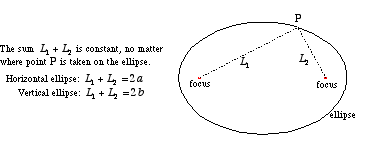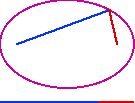# ellipse

Ellipse

A conic section which is essentially a stretchedcircle.

Formally, an ellipse can be defined as follows: For two given points, the foci, an ellipse is the locus of points such that the sum of the distance to each focus is constant. The standard form for the equation of an ellipse is given below.Movie clipEllipse: Sum of distances from the foci is constant (182K)